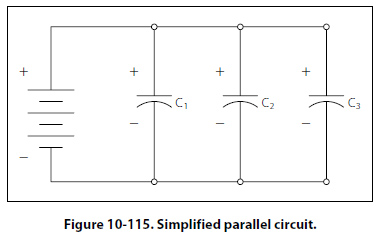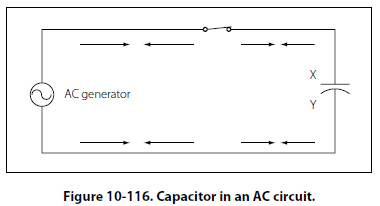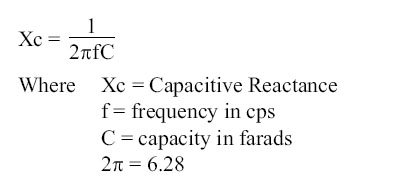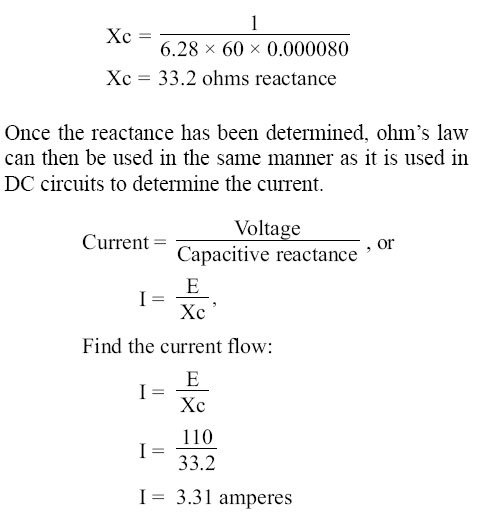Capacitors in Parallel When capacitors are connected in parallel, the effective plate area increases, and the total capacitance is the sum of the individual capacitances. Figure 10-115 shows a simplified parallel circuit.The total charging current from the source divides at the junction of the parallel branches. There is a separate charging current through each branch so that a different charge can be stored by each capacitor. Using Kirchhoff’s current law, the sum of all of the charging currents is then equal to the total current. The sum of the charges (Q) on the capacitors is equal to the total charge. The voltages (E) across all of the parallel branches are equal. With all of this in mind, a general equation for capacitors in parallel can be determined as: QT = Q1 + Q2 + Q3 Because Q = CE: CTET = C1E1 + C2E2 + C3E3 Voltages can be factored out because: ET = E1 + E2 + E3 Leaving us with the equation for capacitors in parallel: CT = C1 + C2 + C3 Consider the following example: If C1 = 330µF, C2 = 220µF Then CT = 330µF + 220µF = 550µF Capacitors in Alternating Current If a source of alternating current is substituted for the battery, the capacitor acts quite differently than it does with direct current. When an alternating current is applied in the circuit, the charge on the plates constantly changes. [Figure 10-116] This means that electricity must flow first from Y clockwise around to X, then from X counterclockwise around to Y, then from Y clockwise around to X, and so on. Although no current flows through the insulator between the plates of the capacitor, it constantly flows in the remainder of the circuit between X and Y. In a circuit in which there is only capacitance, current leads the applied voltage as contrasted with a circuit in which there is inductance, where the current lags the voltage.Capacitive Reactance Xc The effectiveness of a capacitor in allowing an AC flow to pass depends upon the capacitance of the circuit and the applied frequency. To what degree a capacitor allows an AC flow to pass depends largely upon the capacitive value of the capacitor given in farads (f ). The greater the capacitance of the capacitor, the greater the number of electrons, measured in Coulombs, necessary to bring the capacitor to a fully charged state. Once the capacitor approaches or actually reaches a fully charged condition, the polarity of the capacitor will oppose the polarity of the applied voltage, essentially acting then as an open circuit. To further illustrate this characteristic and how it manifests itself in an AC circuit, consider the following. If a capacitor has a large capacitive value, meaning that it requires a relatively large number of electrons to bring it to a fully charged state, then a rather high frequency current can alternate through the capacitor without the capacitor ever reaching a full charge. In this case, if the frequency is high enough and the capacitance large enough that there is never enough time for the capacitor to ever reach a full charge, it is possible that the capacitor may offer very little or no resistance to the current. However, the smaller the capacitance, the fewer electrons are required to bring it up to a full charge and it is more likely that the capacitor will build up enough of an opposing charge that it can present a great deal of resistance to the current if not to the point of behaving like an open circuit. In between these two extreme conditions lies a continuum of possibilities of current opposition depending on the combination of alpplied frequency and the selected capacitance. Current in an AC circuit can be controlled by changing the circuit capacitance in a similar manner that resistance can control the current. The actual AC reactance Xc, which just like resistance, is measured in ohms (O). Capacitive reactance Xc is determined by the following:Sample Problem: A series circuit is assumed in which the impressed voltage is 110 volts at 60 cps, and the capacitance of a condenser is 80 Mf. Find the capacitive reactance and the current flow. Solution: To find capacitive reactance, the equation Xc = 1/(2 pf C) is used. First, the capacitance, 80 Mf, is changed to farads by dividing 80 by 1,000,000, since 1 million microfarads is equal to 1 farad. This quotient equals 0.000080 farad. This is substituted in the equation and©AvStop Online Magazine                                                                                                                                                      Contact Us              Return To Books